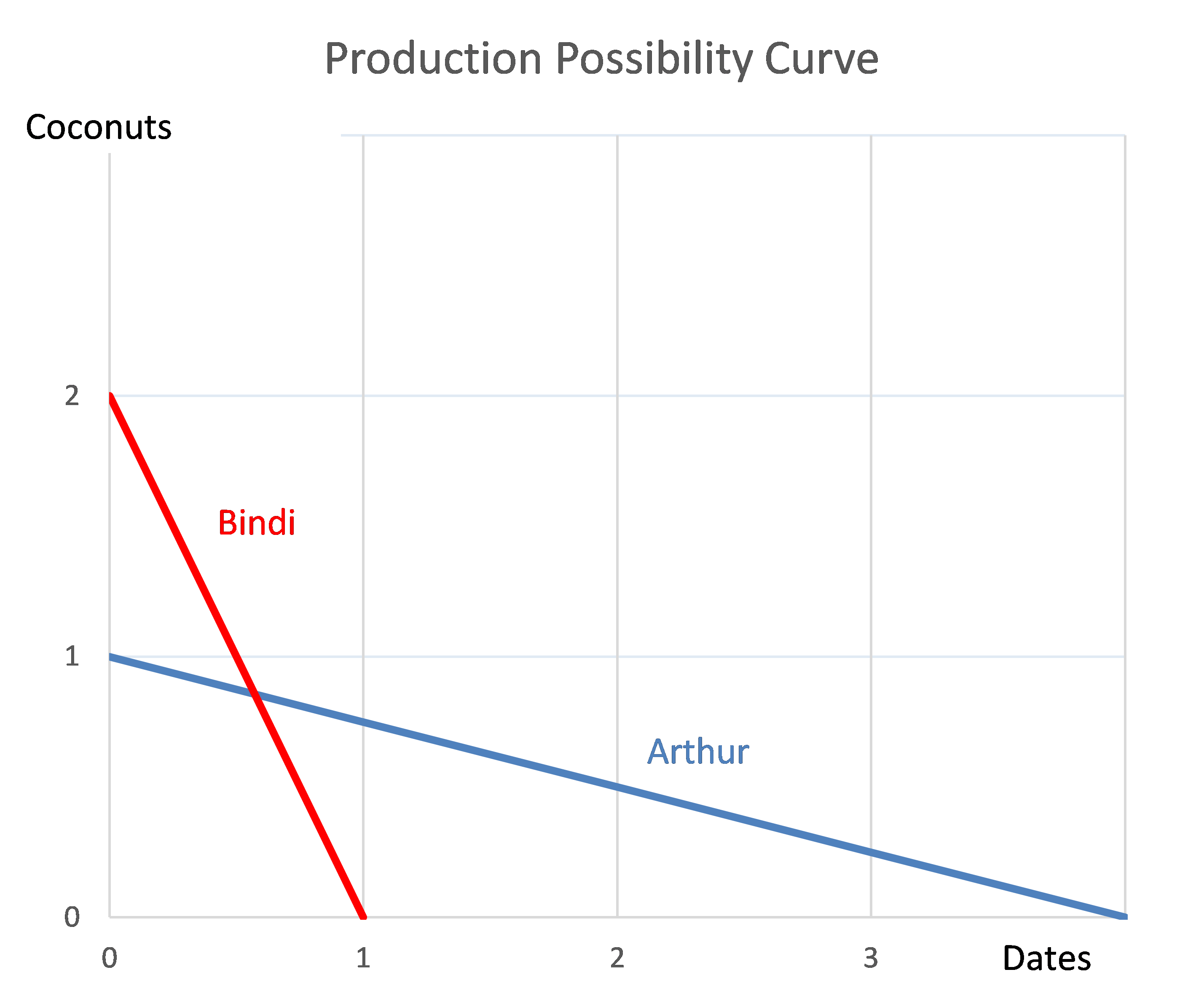# Fight Finance

#### CoursesTagsRandomAllRecentScores

A share just paid its semi-annual dividend of $10. The dividend is expected to grow at 2% every 6 months forever. This 2% growth rate is an effective 6 month rate. Therefore the next dividend will be$10.20 in six months. The required return of the stock 10% pa, given as an effective annual rate.

What is the price of the share now?

For a bond that pays fixed semi-annual coupons, how is the annual coupon rate defined, and how is the bond's annual income yield from time 0 to 1 defined mathematically?

Let: $P_0$ be the bond price now,

$F_T$ be the bond's face value,

$T$ be the bond's maturity in years,

$r_\text{total}$ be the bond's total yield,

$r_\text{income}$ be the bond's income yield,

$r_\text{capital}$ be the bond's capital yield, and

$C_t$ be the bond's coupon at time t in years. So $C_{0.5}$ is the coupon in 6 months, $C_1$ is the coupon in 1 year, and so on.

You have $100,000 in the bank. The bank pays interest at 10% pa, given as an effective annual rate. You wish to consume an equal amount now (t=0) and in one year (t=1) and have nothing left in the bank at the end (t=1). How much can you consume at each time? Estimate the Chinese bank ICBC's share price using a backward-looking price earnings (PE) multiples approach with the following assumptions and figures only. Note that the renminbi (RMB) is the Chinese currency, also known as the yuan (CNY). • The 4 major Chinese banks ICBC, China Construction Bank (CCB), Bank of China (BOC) and Agricultural Bank of China (ABC) are comparable companies; • ICBC 's historical earnings per share (EPS) is RMB 0.74; • CCB's backward-looking PE ratio is 4.59; • BOC 's backward-looking PE ratio is 4.78; • ABC's backward-looking PE ratio is also 4.78; Note: Figures sourced from Google Finance on 25 March 2014. Share prices are from the Shanghai stock exchange. Question 442 economic depreciation, no explanation A fairly valued share's current price is$4 and it has a total required return of 30%. Dividends are paid annually and next year's dividend is expected to be $1. After that, dividends are expected to grow by 5% pa. All rates are effective annual returns. What is the expected dividend cash flow, economic depreciation, and economic income and economic value added (EVA) that will be earned over the second year (from t=1 to t=2) and paid at the end of that year (t=2)? For an asset price to double every 10 years, what must be the expected future capital return, given as an effective annual rate? The below three graphs show probability density functions (PDF) of three different random variables Red, Green and Blue. Let $P_1$ be the unknown price of a stock in one year. $P_1$ is a random variable. Let $P_0 = 1$, so the share price now is$1. This one dollar is a constant, it is not a variable.Which of the below statements is NOT correct? Financial practitioners commonly assume that the shape of the PDF represented in the colour:

All other things remaining equal, a project is worse if its:

Which of the following statements about returns is NOT correct? A stock's:

Arthur and Bindi are the only people on a remote island. Their production possibility curves are shown in the graph.Which of the following statements is NOT correct?